Solving Systems of Equations

Multiple Choice

Identify the choice that best completes the statement or answers the question.

1.        Tell whether the ordered pair (5, –3) is a solution of the system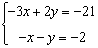.

 a. no b. yes

2.        Solve the system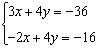by graphing.

3.        The Fun Guys game rental store charges an annual fee of \$5 plus \$5.50 per game rented. The Game Bank charges an annual fee of \$17 plus \$2.50 per game. For how many game rentals will the cost be the same at both stores? What is that cost?

 a. 3 games; \$22 c. 4 games; \$27 b. 2 games; \$16 d. 6 games; \$38

4.        If the pattern in the table continues, in what month will the number of sales of CDs and movie tickets be the same? What number will that be?

 Total Number Sold Month 1 2 3 4 CDs 700 685 670 655 Movie tickets 100 145 190 235

 a. Month 10; 550 c. Month 9; 580 b. Month 11; 550 d. Month 8; 580

5.        Solve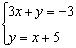by using substitution. Express your answer as an ordered pair.

6.        Solve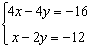by using substitution. Express your answer as an ordered pair.

 a. (8, –4) c. (–2, 4) b. (4, –8) d. (4, 8)

7.        Solve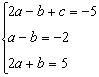by using substitution.

 a.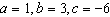c.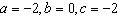b.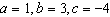d.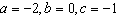8.        Solve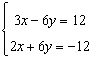by using elimination. Express your answer as an ordered pair.

 a. (–2, –3) c. (0, –2) b. (–2, 0) d. (–8, –6)

9.        Solveby using elimination. Express your answer as an ordered pair.

 a. (5, 0) c. (5, 22.5) b. (5, 17.5) d. (5, 0)

10.        Solve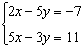by using elimination. Express your answer as an ordered pair.

11.        At the local pet store, zebra fish cost \$2.10 each and neon tetras cost \$1.85 each. If Marsha bought 13 fish for a total cost of \$25.80, not including tax, how many of each type of fish did she buy?

 a. 7 zebra fish, 6 neon tetras c. 6 zebra fish, 7 neon tetras b. 8 zebra fish, 5 neon tetras d. 5 zebra fish, 8 neon tetras

12.        Solve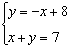13.        Solve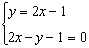.

 a. This system has exactly one solution. b. This system has infinitely many solutions. c. This system has no solution. d. (1, 1) and (0, 0)

14.        Elena and her husband Marc both drive to work. Elena’s car has a current mileage (total distance driven) of 9,000 and she drives 18,000 miles more each year. Marc’s car has a current mileage of 60,000 and he drives 7,000 miles more each year. Will the mileages for the two cars ever be equal? Explain.

 a. No; The equations have different slopes, so the lines do not intersect. b. No; The equations have equal slopes but different y-intercepts, so the lines do not intersect. c. Yes; The equations have different y-intercepts, so the lines intersect. d. Yes; The equations have different slopes, so the lines intersect.

Numeric Response

15.        What value of b will make the system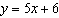andintersect at the point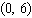?